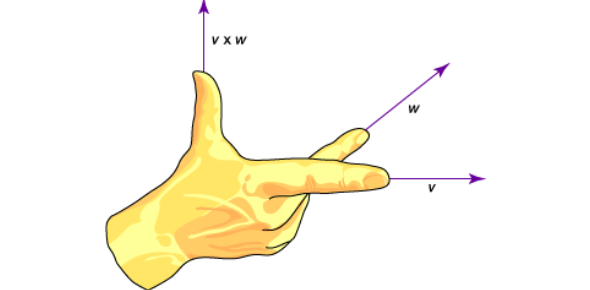# Vector Quiz Questions And Answers

10 Questions | Attempts: 8356
ShareSettingsLet us find out how much you know or understand through these vector quiz questions and answers. Vector is an element of vector space in math and physics. There are many other names that vector has been given. In this quiz, we will be testing your knowledge as well as you will get to learn some more with this interesting quiz. Best of luck to you, and also have fun!

• 1.
Which of these express a vector quantity?
• A.

10kg

• B.

10kg to the north

• C.

10 m/s

• D.

10 m/s to the east

• 2.
Which one of the following is an example of a vector quantity?
• A.

Area

• B.

Mass

• C.

Velocity

• D.

Distance

• 3.
The order in which vectors is added will affect the end result.
• A.

True

• B.

False

• 4.
Vector A is directed northward and vector B is directed eastward. Which of the following vector addition diagrams best represent the addition of vectors A and B and the subsequent resultant?
• A.

A

• B.

B

• C.

C

• D.

D

• E.

E

• F.

F

• 5.
The vector sum (magnitude only) of 67.0 m, north + 38.0 m, south is 7382 m.
• A.

True

• B.

False

• 6.
Vector in physics is the amount of pressure.
• A.

True

• B.

False

• 7.
vector is an object with a magnitude and a direction.
• A.

True

• B.

False

• 8.
When adding vector B to vector A geometrically (or graphically) using the head to the tail method, the resultant is drawn from ____ to the ____.
• A.

Head of A, the tail of B

• B.

Head of B, the tail of A

• C.

The tail of A, head of B a. head of A, tail of B b. tail of A, head of B c. head of B, tail of A tail of A, head of B

• D.

The tail of B, head of A

• 9.
Consider the magnitude and direction of vectors A and B as shown in Diagram 1 above. Which one of the following diagrams would represent B - A = R?
• A.

Diagram A

• B.

Diagram B

• C.

Diagram C

• D.

Diagram D

• 10.
The vector sum (magnitude only) of 25.0 m, north + 18.0 m, East is ___ m.
• A.

7.00

• B.

21.5

• C.

30.8

• D.

35.8

• E.

43.0

• F.

54.2

• G.

949

• H.

None of theseBack to top
×

Wait!
Here's an interesting quiz for you.